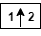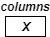# idwt2

Single-level inverse discrete 2-D wavelet transform

## Syntax

``x = idwt2(cA,cH,cV,cD,wname)``
``x = idwt2(cA,cH,cV,cD,LoR,HiR)``
``x = idwt2(___,s)``
``x = idwt2(___,'mode',mode)``
``x = idwt2(cA,[],[],[],___)``
``x = idwt2([],cH,[],[],___)``
``x = idwt2([],[],cV,[],___)``
``x = idwt2([],[],[],cD,___)``

## Description

example

````x = idwt2(cA,cH,cV,cD,wname)` performs a single-level two-dimensional wavelet reconstruction based on the approximation matrix `cA` and details matrices `cH`, `cV`, and `cD` (horizontal, vertical, and diagonal, respectively) using the wavelet specified by `wname`. For additional information, see `dwt2`.Let ```sa = size(cA) = size(cH) = size(cV) = size(cD)```, and let `lf` equal the length of the reconstruction filters associated with `wname`. If the DWT extension mode is set to periodization, the size of `x`, `sx` is equal to `2*sa`. For other extension modes, ```sx = 2*sa-lf+2```. For additional information, see `dwtmode`.```
````x = idwt2(cA,cH,cV,cD,LoR,HiR)` uses the specified lowpass and highpass wavelet reconstruction filters `LoR` and `HiR`, respectively.```
````x = idwt2(___,s)` returns the size-`s` central portion of the reconstruction using any of the previous syntaxes.```
````x = idwt2(___,'mode',mode)` computes the wavelet reconstruction using the specified extension mode `mode`. For additional information, see `dwtmode`. This syntax can be used with any of the previous syntaxes.```
````x = idwt2(cA,[],[],[],___)` returns the single-level reconstructed approximation coefficients matrix `x` based on the approximation coefficients matrix `cA`.```
````x = idwt2([],cH,[],[],___)` returns the single-level reconstructed approximation coefficients matrix `x` based on horizontal detail coefficients matrix `cH`.```
````x = idwt2([],[],cV,[],___)` returns the single-level reconstructed approximation coefficients matrix `x` based on vertical detail coefficients matrix `cV`.```

example

````x = idwt2([],[],[],cD,___)` returns the single-level reconstructed approximation coefficients matrix `x` based on diagonal detail coefficients matrix `cD`.```

## Examples

collapse all

```load woman whos X```
``` Name Size Bytes Class Attributes X 256x256 524288 double ```

The workspace variable `X` contains the image. Perform a single-level wavelet decomposition of `X` use the `db4` wavelet.

`[cA1,cH1,cV1,cD1] = dwt2(X,'db4');`

Invert the decomposition of `X` using the coefficients at level 1.

`A0 = idwt2(cA1,cH1,cV1,cD1,'db4');`

Check for perfect reconstruction.

`max(abs(X(:)-A0(:)))`
```ans = 3.4171e-10 ```

```load tartan imagesc(X) colormap(gray)```Perform a single-level wavelet decomposition using the `db4` wavelet.

`[cA,cH,cV,cD] = dwt2(X,'db4');`

Obtain the wavelet reconstruction using only the diagonal detail coefficients.

`xrecD = idwt2([],[],[],cD,'db4');`

Obtain a second wavelet reconstruction, this time using the horizontal and diagonal detail coefficients.

`xrecHD = idwt2([],cH,[],cD,'db4');`

Display both reconstructions.

```subplot(1,2,1) imagesc(xrecD) title('Diagonal') subplot(1,2,2) imagesc(xrecHD) title('Horizontal-Diagonal') colormap(gray)```## Input Arguments

collapse all

Approximation coefficients, specified as an array. `cA` is expected to be the output of `dwt2`.

Data Types: `double`

Horizontal detail coefficients, specified as an array. `cD` is expected to be the output of `dwt2`.

Data Types: `double`

Vertical detail coefficients, specified as an array. `cV` is expected to be the output of `dwt2`.

Data Types: `double`

Diagonal detail coefficients, specified as an array. `cD` is expected to be the output of `dwt2`.

Data Types: `double`

Wavelet, specified as a character vector or string scalar. `idwt2` supports only orthogonal or biorthogonal wavelets. See `wfilters` for a list of orthogonal and biorthogonal wavelets.

The wavelet specified must be the same wavelet used to obtain the approximation and details coefficients.

Wavelet reconstruction filters, specified as a pair of even-length real-valued vectors. `LoR` is the lowpass reconstruction filter, and `HiR` is the highpass reconstruction filter. The lengths of `LoR` and `HiR` must be equal. See `wfilters` for additional information.

Data Types: `double`

Size of central portion of reconstruction to return, specified as a two element vector of positive integers. `s` must be less than `sx`, the size of `x`.

Data Types: `double`

DWT extension mode used in the wavelet reconstruction, specified as a character vector or string scalar. For possible extension modes, see `dwtmode`.

## Algorithms

The 2-D wavelet reconstruction algorithm for images is similar to the one-dimensional case. The two-dimensional wavelet and scaling functions are obtained by taking the tensor products of the one-dimensional wavelet and scaling functions. This kind of two-dimensional inverse DWT leads to a reconstruction of approximation coefficients at level j from four components: the approximation at level j+1, and the details in three orientations (horizontal, vertical, and diagonal). The following chart describes the basic reconstruction steps for images.where

•— Upsample columns: insert zeros at odd-indexed columns

•— Upsample rows: insert zeros at odd-indexed rows

•— Convolve with filter X the rows of the entry

•— Convolve with filter X the columns of the entry

 Daubechies, Ingrid. Ten Lectures on Wavelets. CBMS-NSF Regional Conference Series in Applied Mathematics 61. Philadelphia, Pa: Society for Industrial and Applied Mathematics, 1992.

 Mallat, S.G. “A Theory for Multiresolution Signal Decomposition: The Wavelet Representation.” IEEE Transactions on Pattern Analysis and Machine Intelligence 11, no. 7 (July 1989): 674–93. https://doi.org/10.1109/34.192463.

 Meyer, Y. Wavelets and Operators. Translated by D. H. Salinger. Cambridge, UK: Cambridge University Press, 1995.